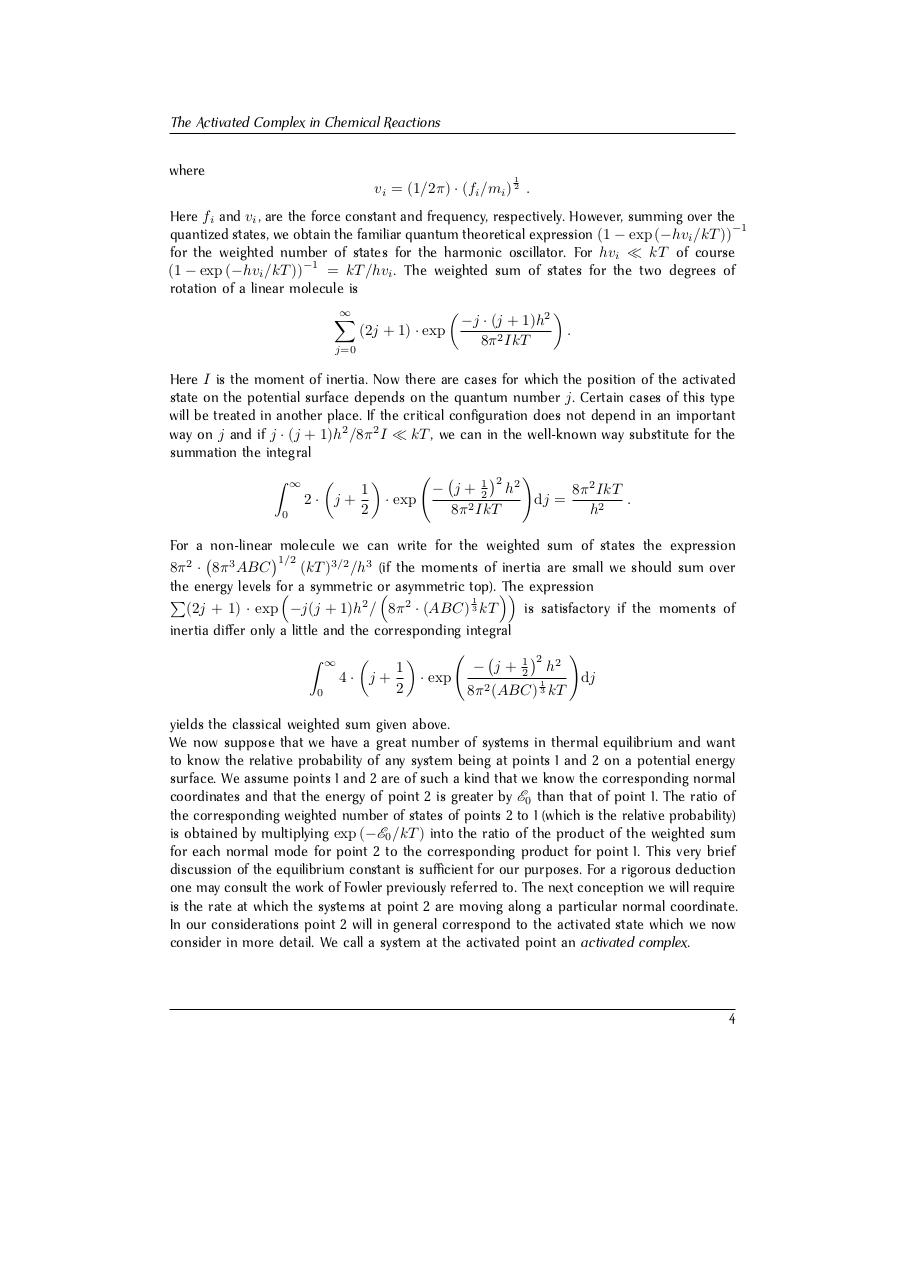# Henry Eyring. The Activated Complex in Chemi.pdfPage 1 2 3 4 5 6 7 8 9 10 11 12 13

#### Text preview

The Activated Complex in Chemical Reactions
where

1

vi = (1/2π) · (fi /mi ) 2 .
Here fi and vi , are the force constant and frequency, respectively. However, summing over the
−1
quantized states, we obtain the familiar quantum theoretical expression (1 − exp (−hvi /kT ))
for the weighted number of states for the harmonic oscillator. For hvi  kT of course
−1
(1 − exp (−hvi /kT ))
= kT /hvi . The weighted sum of states for the two degrees of
rotation of a linear molecule is



X
−j · (j + 1)h2
(2j + 1) · exp
.
8π 2 IkT
j=0
Here I is the moment of inertia. Now there are cases for which the position of the activated
state on the potential surface depends on the quantum number j. Certain cases of this type
will be treated in another place. If the critical configuration does not depend in an important
way on j and if j · (j + 1)h2 /8π 2 I  kT , we can in the well-known way substitute for the
summation the integral
2 !

Z ∞ 
− j + 12 h2
1
8π 2 IkT
· exp
2· j+
dj =
.
2
2
8π IkT
h2
0
For a non-linear molecule we can write for the weighted sum of states the expression
1/2
8π 2 · 8π 3 ABC
(kT )3/2 /h3 (if the moments of inertia are small we should sum over
the energy levels for
The expression

 a symmetric or
 asymmetric top).
P
1
(2j + 1) · exp −j(j + 1)h2 / 8π 2 · (ABC) 3 kT
is satisfactory if the moments of
inertia differ only a little and the corresponding integral
!
2

Z ∞ 
− j + 21 h2
1
4· j+
· exp
dj
1
2
8π 2 (ABC) 3 kT
0
yields the classical weighted sum given above.
We now suppose that we have a great number of systems in thermal equilibrium and want
to know the relative probability of any system being at points 1 and 2 on a potential energy
surface. We assume points 1 and 2 are of such a kind that we know the corresponding normal
coordinates and that the energy of point 2 is greater by E0 than that of point 1. The ratio of
the corresponding weighted number of states of points 2 to 1 (which is the relative probability)
is obtained by multiplying exp (−E0 /kT ) into the ratio of the product of the weighted sum
for each normal mode for point 2 to the corresponding product for point 1. This very brief
discussion of the equilibrium constant is sufficient for our purposes. For a rigorous deduction
one may consult the work of Fowler previously referred to. The next conception we will require
is the rate at which the systems at point 2 are moving along a particular normal coordinate.
In our considerations point 2 will in general correspond to the activated state which we now
consider in more detail. We call a system at the activated point an activated complex.

4